Author: Oscar Cronquist Article last updated on March 23, 2023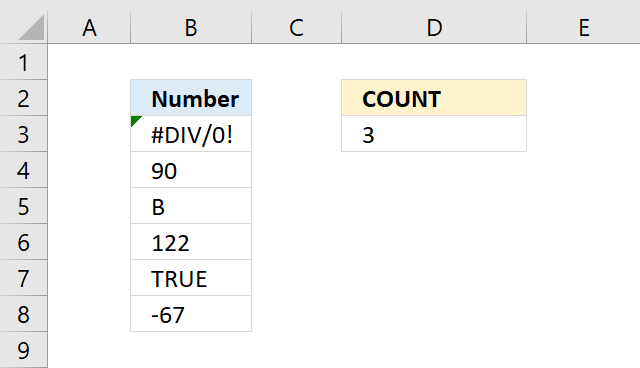The COUNT function counts all numerical values in an argument, it allows you to have up to 255 arguments.

Formula in cell D3:

=COUNT(B3:B8)

Blank cells, boolean values and text values are not counted. It differs from most other functions, ignoring error values.

## 1. COUNT Function Syntax

COUNT(value1, [value2], ...)

## 2. COUNT Function Arguments

 value1 Required. A cell reference, array, or constants for which you want to count numbers. [value2] Optional. Up to 255 additional arguments.

## 3. COUNT function - exampleFormula in cell D3:

=COUNT(B3:B8)

### 3.1 Explaining formula

COUNT(B3:B8)

becomes

COUNT({#DIV/0!;90;"B";122;TRUE;-67})

and returns 3. There are three numbers in cell range B3:B8, they are 90, 122, and -67.

## 4. COUNT function - count numbers in an array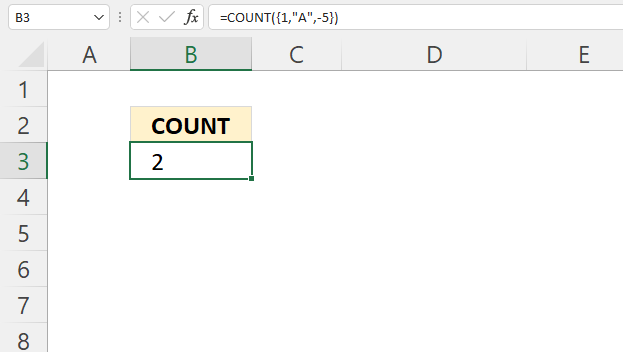The COUNT function works fine with constants in an array as well.

Formula in cell B3:

=COUNT({1,"A",-5})

There are two numbers in {1,"A",-5}, they are 1 and -5.

## 5. COUNT function - count digits in a cell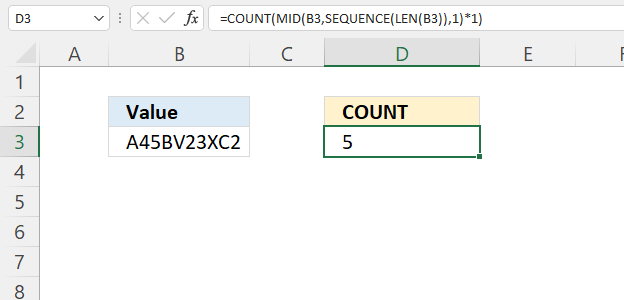Formula in cell D3:

=COUNT(MID(B3,SEQUENCE(LEN(B3)),1)*1)

### 5.1 Explaining formula

#### Step 1 - Count characters in cell

The LEN function returns the number of characters in a cell value.

LEN(text)

LEN(B3)

becomes

LEN("A45BV23XC2")

and returns 10. There are ten characters in cell B3.

#### Step 2 - Create numbers from 1 to n

The SEQUENCE function creates a list of sequential numbers.

SEQUENCE(rows, [columns], [start], [step])

SEQUENCE(LEN(B3))

becomes

SEQUENCE(10)

and returns

{1; 2; 3; 4; 5; 6; 7; 8; 9; 10}.

#### Step 3 - Split characters into an array

The MID function returns a substring from a string based on the starting position and the number of characters you want to extract.

MID(textstart_numnum_chars)

MID(B3,SEQUENCE(LEN(B3)),1)

becomes

MID(B3,{1; 2; 3; 4; 5; 6; 7; 8; 9; 10},1)

becomes

MID("A45BV23XC2",{1; 2; 3; 4; 5; 6; 7; 8; 9; 10},1)

and returns

{"A"; "4"; "5"; "B"; "V"; "2"; "3"; "X"; "C"; "2"}.

#### Step 4 - Convert text numbers to numbers

The asterisk lets you multiply numbers in an Excel formula, it also lets you convert "text" numbers to regular numbers.

MID(B3,SEQUENCE(LEN(B3)),1)*1

becomes

{"A"; "4"; "5"; "B"; "V"; "2"; "3"; "X"; "C"; "2"}*1

and returns

{#VALUE!; 4; 5; #VALUE!; #VALUE!; 2; 3; #VALUE!; #VALUE!; 2}.

Note the numbers are not enclosed by double quotes any more. The text values are now error values but that changes nothing, the COUNT function ignores error values.

#### Step 5 - Count numbers in array

COUNT(MID(B3,SEQUENCE(LEN(B3)),1)*1)

becomes

COUNT({#VALUE!; 4; 5; #VALUE!; #VALUE!; 2; 3; #VALUE!; #VALUE!; 2})

and returns 5.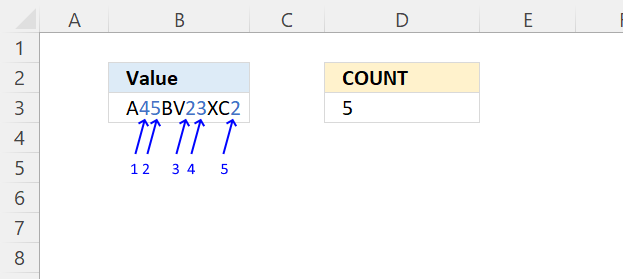## 6. COUNT function - count digits in a cell range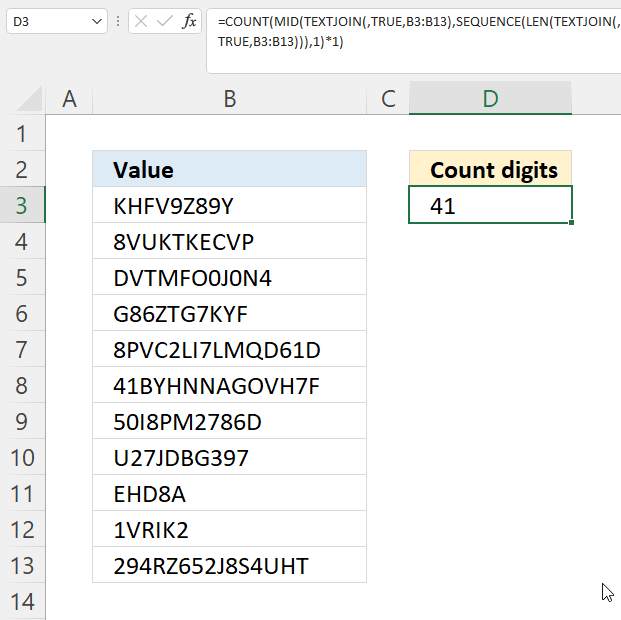Formula in cell D3:

=COUNT(MID(TEXTJOIN(, TRUE, B3:B13), SEQUENCE(LEN(TEXTJOIN(, TRUE, B3:B13))), 1)*1)

### 6.1 Explaining formula

#### Step 1 - Join values in cell range B3:B13

The TEXTJOIN function combines text strings from multiple cell ranges and also delimiting characters.

TEXTJOIN(delimiterignore_emptytext1[text2], ...)

TEXTJOIN(, TRUE, B3:B13)

becomes

TEXTJOIN(, TRUE, {"KHFV9Z89Y"; "8VUKTKECVP"; "DVTMFO0J0N4"; "G86ZTG7KYF"; "8PVC2LI7LMQD61D"; "41BYHNNAGOVH7F"; "50I8PM2786D"; "U27JDBG397"; "EHD8A"; "1VRIK2"; "294RZ652J8S4UHT"})

and returns

"KHFV...UHT".

#### Step 2 - Count characters in string

The LEN function returns the number of characters in a cell value.

LEN(text)

LEN(TEXTJOIN(, TRUE, B3:B13))

becomes

LEN("KHFV...UHT")

and returns 116. There is a total of 116 characters in the string.

#### Step 3 - Create numbers from 1 to n

The SEQUENCE function creates a list of sequential numbers.

SEQUENCE(rows, [columns], [start], [step])

SEQUENCE(LEN(TEXTJOIN(, TRUE, B3:B13)))

becomes

SEQUENCE(116)

and returns {1;2;3;...;116}.

#### Step 4 - Split string

The MID function returns a substring from a string based on the starting position and the number of characters you want to extract.

MID(textstart_numnum_chars)

MID(TEXTJOIN(, TRUE, B3:B13), SEQUENCE(LEN(TEXTJOIN(, TRUE, B3:B13))), 1)

becomes

MID("KHFV...UHT", {1;2;3;...;116}, 1)

and returns

{"K";"H";"F";...;"T"}

#### Step 5 - Convert text numbers to regular numbers

The asterisk lets you multiply numbers in an Excel formula, it also lets you convert "text" numbers to regular numbers.

MID(TEXTJOIN(, TRUE, B3:B13), SEQUENCE(LEN(TEXTJOIN(, TRUE, B3:B13))), 1)*1

becomes

{"K";"H";"F";...;"T"}*1

and returns

{#VALUE!;#VALUE!;#VALUE!;...;#VALUE!}

#### Step 6 - Count numbers

COUNT(MID(TEXTJOIN(, TRUE, B3:B13), SEQUENCE(LEN(TEXTJOIN(, TRUE, B3:B13))), 1)*1)

becomes

COUNT({#VALUE!;#VALUE!;#VALUE!;...;#VALUE!})

and returns 41. There are 41 digits in cell range B3:B13.

## 7. COUNT function - count negative numbers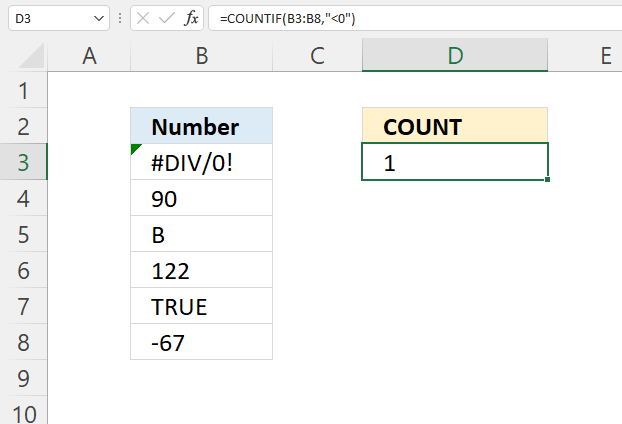Formula in cell D3:

=COUNTIF(B3:B8, "<0")

### 7.1 Explaining formula

The COUNTIF function calculates the number of cells that meet a condition.

COUNTIF(rangecriteria)

COUNTIF(B3:B8, "<0")

becomes

COUNTIF({#DIV/0!;90;"B";122;TRUE;-67}, "<0")

and returns 1. -67 is the only negative number in the array.

## 8. COUNT function - count positive numbers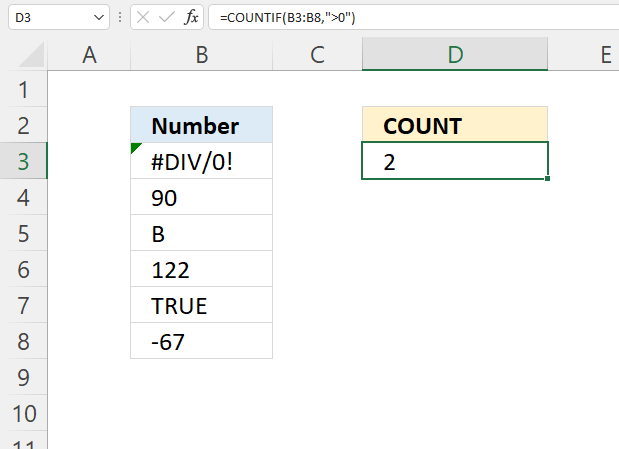Formula in cell D3:

=COUNTIF(B3:B8, ">0")

### 8.1 Explaining formula

The COUNTIF function calculates the number of cells that meet a condition.

COUNTIF(rangecriteria)

COUNTIF(B3:B8, ">0")

becomes

COUNTIF({#DIV/0!;90;"B";122;TRUE;-67}, ">0")

and returns 2. 90 and 122 are larger than 0 (zero).

## 9. COUNT function - count numbers in a given range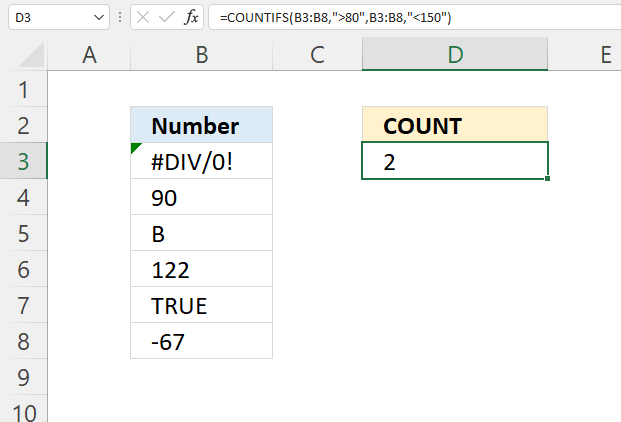Formula in cell D3:

=COUNTIFS(B3:B8, ">80",B3:B8, "<150")

### 9.1 Explaining formula

The COUNTIFS function calculates the number of cells across multiple ranges that equals all given conditions.

COUNTIFS(criteria_range1criteria1, [criteria_range2criteria2]…)

COUNTIFS(B3:B8, ">80",B3:B8, "<150")

becomes

COUNTIFS({#DIV/0!;90;"B";122;TRUE;-67}, ">80",{#DIV/0!;90;"B";122;TRUE;-67}, "<150")

and returns 2. 90 and 122 are larger than 80 and smaller than 150.

## 10. Count numbers per row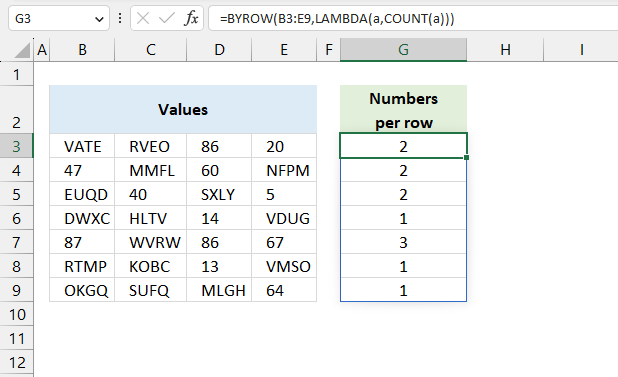The image above shows an Excel 365 dynamic array formula that calculates how many numbers there are on each row in cell range B3:E9.

Excel 365 formula in cell G3:

=BYROW(B3:E9,LAMBDA(a,COUNT(a)))

### Explaining formula

#### Step 1 - Count cells only numbers

The COUNT function counts all numerical values in an argument.

Function syntax: COUNT(value1, [value2], ...)

COUNT(a)

#### Step 2 - Build the LAMBDA function

The LAMBDA function build custom functions without VBA, macros or javascript.

Function syntax: LAMBDA([parameter1, parameter2, …,] calculation)

LAMBDA(a,COUNT(a))

#### Step 3 - Count nonempty cells per row

The BYROW function puts values from an array into a LAMBDA function row-wise.

Function syntax: BYROW(array, lambda(array, calculation))

BYROW(B3:E9,LAMBDA(a,COUNT(a)))

## 11. Sort rows based on numbers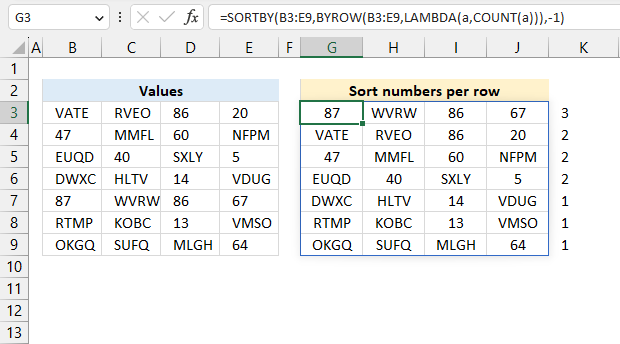Excel 365 dynamic array formula in cell G3:

=SORTBY(B3:E9,BYROW(B3:E9,LAMBDA(a,COUNT(a))),-1)

### Explaining formula

This formula works just like the example in section 10, but it also sorts rows based on the count of cells containing only numbers. The values in G3:J3 has three numbers, 87, 86, and 67. That row has the largest number of numerical values in B3:E9 shown in cell K3.

The SORTBY function sorts a cell range or array based on values in a corresponding range or array.

Function syntax: SORTBY(array, by_array1, [sort_order1], [by_array2, sort_order2],…)

## 12. Count numbers per column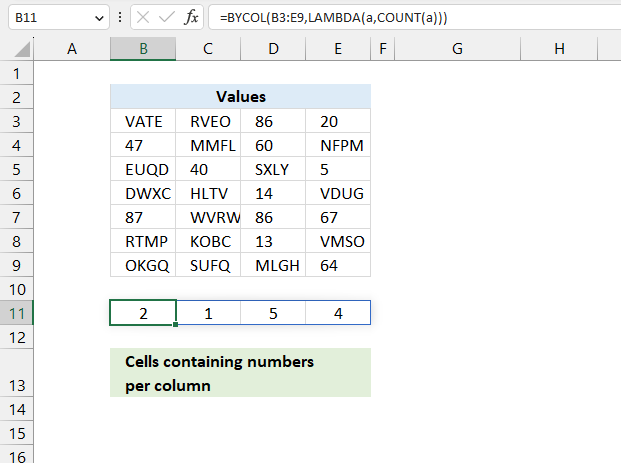The image above shows an Excel 365 dynamic array formula that counts numbers per column in cell range B3:E9.

Excel 365 formula in cell B11:

=BYCOL(B3:E9,LAMBDA(a,COUNT(a)))

### Explaining formula

#### Step 1 - Count numbers

The COUNT function counts all numerical values in an argument.

Function syntax: COUNT(value1, [value2], ...)

COUNT(a)

#### Step 2 - Build the LAMBDA function

The LAMBDA function build custom functions without VBA, macros or javascript.

Function syntax: LAMBDA([parameter1, parameter2, …,] calculation)

LAMBDA(a,COUNT(a))

#### Step 3 - Count numbers per column

The BYCOL function passes all values in a column based on an array to a LAMBDA function, the LAMBDA function calculates new values based on a formula you specify. It then continues with the next column until all columns in the array have been processed.

Function syntax: BYCOL(array, lambda(array, calculation))

BYCOL(B3:E9,LAMBDA(a,COUNT(a)))

## 13. Sort columns based on numbers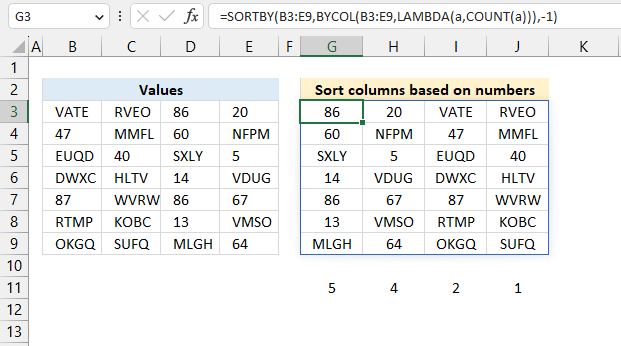Excel 365 dynamic array formula in cell G3:

=SORTBY(B3:E9,BYCOL(B3:E9,LAMBDA(a,COUNT(a))),-1)

### Explaining formula

This formula works just like the example in section 12, however, it also sorts the columns based on the number of cells containing only numbers.

The image above shows 5 in cell G11, and there are five numbers cells in G3:G9. The next cell H11 displays 4, there are four cells in H3:H9 containing only numbers.

The SORTBY function sorts a cell range or array based on values in a corresponding range or array.

Function syntax: SORTBY(array, by_array1, [sort_order1], [by_array2, sort_order2],…)